2018-6-28 22:59
5400

# CONDITIONAL EXECUTION 条件执行指令

We already briefly touched the conditions’ topic while discussing the CPSR register. We use conditions for controlling the program’s flow during it’s runtime usually by making jumps (branches) or executing some instruction only when a condition is met. The condition is described as the state of a specific bit in the CPSR register. Those bits change from time to time based on the outcome of some instructions. For example, when we compare two numbers and they turn out to be equal, we trigger the Zero bit (Z = 1), because under the hood the following happens: a – b = 0. In this case we have equal condition. If the first number was bigger, we would have a GreaterThan condition and in the opposite case –LowerThan. There are more conditions, like Lower orEqual (LE),Greater orEqual (GE) and so on.

The following table lists the available condition codes, their meanings, and the status of the flags that are tested.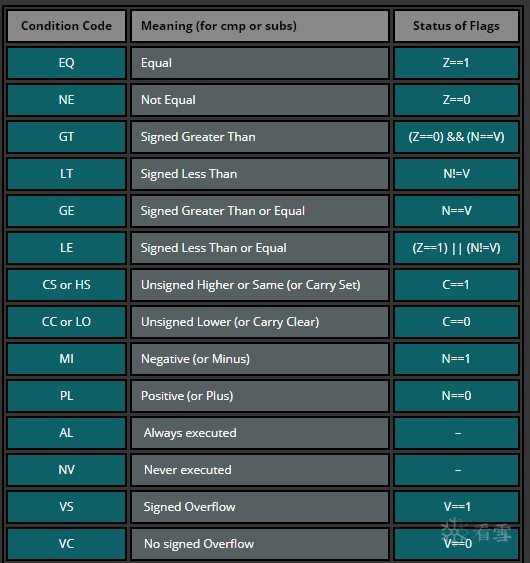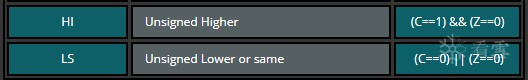We can use the following piece of code to look into a practical use case of conditions where we perform conditional addition.

```.global main

main:
mov     r0, #2     /* setting up initial variable */
cmp     r0, #3     /* comparing r0 to number 3. Negative bit get's set to 1 R0与数字3比较，N位置一*/
addlt   r0, r0, #1 /* increasing r0 IF it was determined that it is smaller (lower than) number 3 如果R0比3小就将R0自增1*/
cmp     r0, #3     /* comparing r0 to number 3 again. Zero bit gets set to 1. Negative bit is set to 0 再次比较r0和3，Z标志位置位 */
addlt   r0, r0, #1 /* increasing r0 IF it was determined that it is smaller (lower than) number 3 如果r0小于3给r0自增1*/
bx      lr```

The first CMP instruction in the code above triggers Negative bit to be set (2 – 3 = -1) indicating that the value in r0 is Lower Than number 3. Subsequently, the ADDLT instruction is executed because LT condition is full filled when V != N (values of overflow and negative bits in the CPSR are different). Before we execute second CMP, our r0 = 3. That’s why second CMP clears out Negative bit (because 3 – 3 = 0, no need to set the negative flag) and sets theZero flag (Z = 1). Now we have V = 0 and N = 0 which results in LT condition to fail. As a result, the second ADDLT is not executed and r0 remains unmodified. The program exits with the result 3.

# CONDITIONAL EXECUTION IN THUMB Thumb模式下的条件执行

In the Instruction Set chapter we talked about the fact that there are different Thumb versions. Specifically, the Thumb version which allows conditional execution (Thumb-2). Some ARM processor versions support the “IT” instruction that allows up to 4 instructions to be executed conditionally in Thumb state.

Syntax: IT{x{y{z}}}cond    语法结构： IT{x{y{z}}} cond（译者注：xyz指IT后最多再跟三个大写字母，大写字母可以是T，可以是E，T就是then，E就是else）

cond specifies the condition for the first instruction in the IT block

cond规定了执行IT语句块里的第一条指令需要满足的条件

x specifies the condition switch for the second instruction in the IT block

x规定了执行的IT语句块中第二条指令需要满足的条件

y specifies the condition switch for the third instruction in the IT block

y规定了执行IT语句块里的第三条指令需要满足的条件

z specifies the condition switch for the fourth instruction in the IT block

z 规定了执行IT语句块里的第四条指令需要满足的条件

The structure of the IT instruction is “IF-Then-(Else)” and the syntax is a construct of the two letters T and E:

IT指令集的结构是： “IF-Then-(Else)”，它的语法结构由两个字母构成：

IT refers to If-Then (next instruction is conditional)

IT代表If-Then（下一条指令是条件指令）

ITT refers to If-Then-Then (next 2 instructions are conditional)

ITT代表If-Then-Then（接下来的两条指令是条件指令）

ITE refers to If-Then-Else (next 2 instructions are conditional)

ITE代表 If-Then-Else（接下来的两条指令是条件指令）

ITTE refers to If-Then-Then-Else (next 3 instructions are conditional)

ITTE代表 If-Then-Then-Else （接下来的三条指令是条件指令）

ITTEE refers to If-Then-Then-Else-Else (next 4 instructions are conditional)

ITTEE代表 If-Then-Then-Else-Else （接下来的四条指令是条件指令）

Each instruction inside the IT block must specify a condition suffix that is either the same or logical inverse. This means that if you use ITE, the first and second instruction (If-Then) must have the same condition suffix and the third (Else) must have the logical inverse of the first two. Here are some examples from the ARM reference manual which illustrates this logic:

IT语句块内的每个指令必须指定一个条件后缀，该条件后缀那么相同要么在逻辑上相反。这意味着，如果使用了ITE，第一和第二指令（If-Then）必须具有相同的条件后缀，而第三条指令（else语句）必须和前面两条语句逻辑相反。以下是ARM参考手册中的一些例子，说明了这一逻辑：

```ITTE   NE           ; Next 3 instructions are conditional 接下来的三条指令是条件指令
ANDNE  R0, R0, R1   ; ANDNE does not update condition flags ANDNE不更新条件标志位
MOVEQ  R2, R3       ; Conditional move 条件赋值指令

ITE    GT           ; Next 2 instructions are conditional    下面两条指令是条件指令
ADDGT  R1, R0, #55  ; Conditional addition in case the GT is true  如果GT为1执行条件的相加指令
ADDLE  R1, R0, #48  ; Conditional addition in case the GT is not true   如果GT为0执行的条件相加指令

ITTEE  EQ           ; Next 4 instructions are conditional下面四条指令是条件指令
MOVEQ  R0, R1       ; Conditional MOV   条件赋值指令
ANDNE  R3, R3, #1   ; Conditional AND  条件与指令
BNE.W  dloop        ; Branch instruction can only be used in the last instruction of an IT block  条件分支指令只能用于IT语句块的结尾```

Wrong syntax:错误的语法：

```IT     NE           ; Next instruction is conditional    下面一条不是条件执行指令
ADD    R0, R0, R1   ; Syntax error: no condition code used in IT block.  语法错误：IT语句块中没有使用条件执行指令```

Here are the conditional codes and their opposite: 以下总结了条件指令和逻辑相反的指令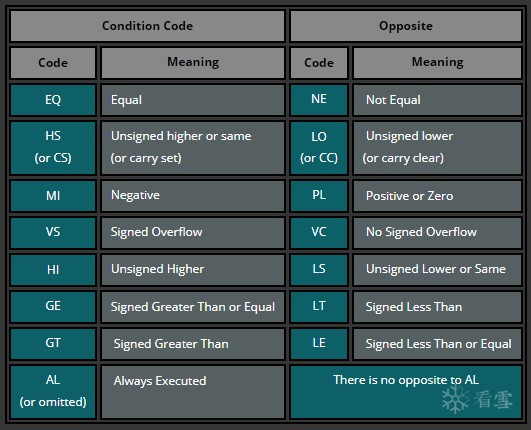Let’s try this out with the following example code:用下面的示例代码尝试一下

```.syntax unified    @ this is important!这句话很关键
.text
.global _start

_start:
.code 32
add r3, pc, #1   @ increase value of PC by 1 and add it to R3
bx r3            @ branch + exchange to the address in R3 -> switch to Thumb state because LSB = 1

.code 16         @ Thumb state
cmp r0, #10
ite eq           @ if R0 is equal 10...
addeq r1, #2     @ ... then R1 = R1 + 2
addne r1, #3     @ ... else R1 = R1 + 3
bkpt```

.code 32

This example code starts in ARM state. The first instruction adds the address specified in PC plus 1 to R3 and then branches to the address in R3.  This will cause a switch to Thumb state, because the LSB (least significant bit) is 1 and therefore not 4 byte aligned. It’s important to use bx (branch + exchange) for this purpose. After the branch the T (Thumb) flag is set and we are in Thumb state.

.32

.code 16

In Thumb state we first compare R0 with #10, which will set the Negative flag (0 – 10 = – 10). Then we use an If-Then-Else block. This block will skip the ADDEQ instruction because the Z (Zero) flag is not set and will execute the ADDNE instruction because the result was NE (not equal) to 10.

Stepping through this code in GDB will mess up the result, because you would execute both instructions in the ITE block. However running the code in GDB without setting a breakpoint and stepping through each instruction will yield to the correct result setting R1 = 3.

# BRANCHES 分支

Branches (aka Jumps) allow us to jump to another code segment. This is useful when we need to skip (or repeat) blocks of codes or jump to a specific function. Best examples of such a use case are IFs and Loops. So let’s look into the IF case first.

```.global main

main:
mov     r1, #2     /* setting up initial variable a 给变量a赋初始值*/
mov     r2, #3     /* setting up initial variable b 给变量b赋初始值*/
cmp     r1, r2     /* comparing variables to determine which is bigger  比较两个变量看看谁更大*/
blt     r1_lower   /* jump to r1_lower in case r2 is bigger (N==1)  如果r2更大就跳转到r1_lower */
mov     r0, r1     /* if branching/jumping did not occur, r1 is bigger (or the same) so store r1 into r0  如果分支/跳转没有发生，r1大于或者等于r0，就将r1的值赋值给r0*/
b       end        /* proceed to the end 跳到end去处理 */
r1_lower:
mov r0, r2         /* We ended up here because r1 was smaller than r2, so move r2 into r0 */
b end              /* proceed to the end 跳到end去处理 */
end:
bx lr              /* THE END */```

The code above simply checks which of the initial numbers is bigger and returns it as an exit code. A C-like pseudo-code would look like this:

```int main() {
int max = 0;
int a = 2;
int b = 3;
if(a < b) {
max = b;
}
else {
max = a;
}
return max;
}```

Now here is how we can use conditional and unconditional branches to create a loop.

```.global main

main:
mov     r0, #0     /* setting up initial variable a */
loop:
cmp     r0, #4     /* checking if a==4 */
beq     end        /* proceeding to the end if a==4 */
add     r0, r0, #1 /* increasing a by 1 if the jump to the end did not occur */
b loop             /* repeating the loop */
end:
bx lr              /* THE END */```

A C-like pseudo-code of such a loop would look like this:

```int main() {
int a = 0;
while(a < 4) {
a= a+1;
}
return a;
}```

## b,bx,blx

There are three types of branching instructions:有三类分支指令。他们分别是：

1.Branch (B)   分支指令（B）

a)Saves (PC+4) in LR and jumps to function

3.Branch exchange (BX) and Branch link exchange (BLX)  BX指令：分支切换指令和BLX（分支连接切换指令）

a)Same as B/BL + exchange instruction set (ARM <-> Thumb)

b)Needs a register as first operand: BX/BLX reg

BX/BLX is used to exchange the instruction set from ARM to Thumb.

BX/BLX用来从ARM指令集切换到Thumb指令集

```.text
.global _start

_start:
.code 32         @ ARM mode
add r2, pc, #1   @ put PC+1 into R2
bx r2            @ branch + exchange to R2

.code 16          @ Thumb mode
mov r0, #1```

The trick here is to take the current value of the actual PC, increase it by 1, store the result to a register, and branch (+exchange) to that register. We see that the addition (add r2, pc, #1) will simply take the effective PC address (which is the current PC register’s value + 8 -> 0x805C) and add 1 to it (0x805C + 1 = 0x805D). Then, the exchange happens if the Least Significant Bit (LSB) of the address we branch to is 1 (which is the case, because 0x805D = 10000000 01011101), meaning the address is not 4 byte aligned. Branching to such an address won’t cause any misalignment issues. This is how it would look like in GDB (with GEF extension):Please note that the GIF above was created using the older version of GEF so it’s very likely that you see a slightly different UI and different offsets. Nevertheless, the logic is the same.

### Conditional Branches条件分支指令

Branches can also be executed conditionally and used for branching to a function if a specific condition is met. Let’s look at a very simple example of a conditional branch suing BEQ. This piece of assembly does nothing interesting other than moving values into registers and branching to another function if a register is equal to a specified value.

```.text
.global _start

_start:
mov r0, #2
mov r1, #2
cmp r0, #4
beq func1
b func2
func1:
mov r1, r0
bx  lr
func2:
mov r0, r1
bx  lr```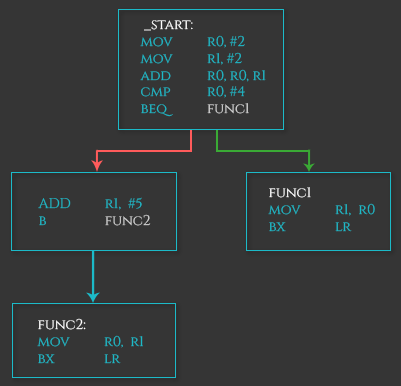CTF训练营-Web篇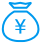打赏次数 1 雪花 + 5.00junkboy 5 2018/06/29